## Pioneers Anomaly: Deep Space, ANRI-Effect

Khavroshkin OB

Institute of Earth physics. RAS, Moscow, Russia

Tsyplakov VV

Institute of Earth physics. RAS, Moscow, Russia

Georgievich ST

Doctor of Technical Sciences, Professor, General Director LLC «Nauchtekhlitizdat» 107258, Russian Federation, Moscow

Article
Article Info
Author Info
Figures & Data

## Abstract

The problem of the cause of weak braking of the "Pioner10" and "Pioner11" spacecraft is investigated. Contrary to the established ideas, the usual modern physical mechanism was found. The mechanism is not a deceleration, but a weakening of a very small kind of thrust due to the interaction of the solar neutrino stream with radioisotope energy sources installed on the "Pioneers". This interaction has been studied on Earth and is known as the Anomalous Neutrino Radio - Isotope (ANRI) absorption or effect.

## Keywords

Pioneers; Radioisotope Energy Sources; Solar Neutrino Flux; ANRI Effect

## Introduction

According to many foreign scientists, deep space flights will bring riddles, the quick solution of which is problematic. However, this is not indisputable, as well as the concept of deep space, the boundaries of which should be drawn behind the Oort cloud. Probably, first it is necessary to study more deeply and in detail on Earth those physical effects and processes, the manifestation of which should be expected in space. So, let us consider the features of the flight trajectories of the spacecraft "Pioneer-10" and "Pioneer-11", which successfully passed the orbits of giant planets, but have unforeseen anomalies already at the border of the solar system (Figure. 1). Much of the Pioneer evidence comes from Naked Science (Internet). For the probes Pioneer 10 (launched in March 1972) and Pioneer 11 (April 1973), an anomaly in their flight trajectory was first discovered in the 1980s, when their main mission had already been completed.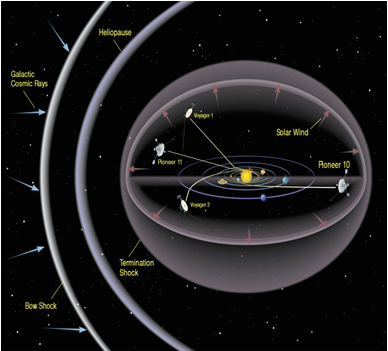Figure 1. Scheme the Exit of "Pioneers" and "Voyagers" Outside the Solar System

The devices move in free flight in different ways: "Pioneer-10" to the edge of the Milky Way, in the direction of the constellation Taurus; Pioneer 11, on the other hand, is directed towards the center of the Galaxy, in the direction of the Shield constellation. Only the previously obtained acceleration and external forces affect the flight of spacecraft. Forces are gravitational and non-gravitational. All forces that can affect the flight of spacecraft have been identified and taken into account by a team of specialists. The problem is created by one unknown and incomprehensible force that pulls the probes back and is the mystery of the Pioneers. The power is negligible, but it is there. The latest calculations, obtained by 2002, say that the magnitude of the inexplicable negative acceleration is (8.74 ± 1.33) • 10-10 m / s2. This is negligible, but has already led to a deviation of the vehicles by about 400 thousand kilometers from the calculated trajectory. It would seem that the probes have flown billions of kilometers. At the time of the loss of communication with "Pioneer-10" (January 23, 2003), it had moved away from the Earth by more than 12 billion kilometers. These are 82 astronomical units, that is, 82 distances from the Earth to the Sun. Communication with "Pioneer-11" was lost on September 30, 1995, the device was already at a distance of 6.5 billion kilometers from the Sun, or 43 AU. e. With such a slight deviation or anomaly for Western experts, they may indicate the presence of something significant, but not yet discovered. Moreover, in astrophysics. (True, in Russia there is a well-founded effect that removes all mystery. See below). It is important that only the Pioneers, unlike other vehicles, are in flight without additional course corrections for a long time. Note that in search of the cause of the "Pioneers" anomaly, many hypotheses were considered, but after careful analysis all of them turned out to be unacceptable. As is known, radioisotope thermoelectric generators using plutonium-238 are installed on board the vehicles as energy sources (Figure. 2).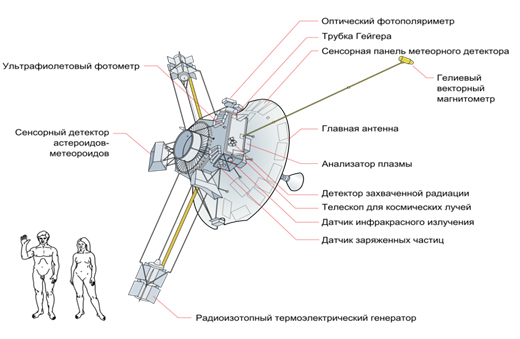Figure 2.  Diagram of the "Pioneer-10" apparatus / © wikipedia.org

Radioisotope generators use thermal energy, which is released during the natural decay of radioactive isotopes, and with the help of a thermoelectric generator convert it into electricity. Plutonium-238 is such a radioactive isotope whose decay powers the equipment on board the probes. Each probe has four generators, which are fixed on two three-meter outrigger rods, away from the scientific instruments of the apparatus. Each probe has four generators, which are fixed at the ends of two three-meter outrigger rods, at a distance from the scientific instruments of the apparatus.

#### About braking mechanisms

Initially, a group of researchers (NASA) managed to build a mathematical model that explains the anomalous acceleration of the "Pioneers" by at least 70%. In their opinion, the whole point is in the heat fluxes coming from the probe in different directions. And the main source of heat is radioisotope generators, which supplied energy to the onboard equipment (Fig. 2). In flight, the probes are stabilized by rotating around the longitudinal axis. The heat radiated perpendicular to the longitudinal axis is dissipated in all directions evenly due to the rotation of the apparatus around the longitudinal axis, and does not affect the movement of the probes. Calculations have shown that the heat flux emitted in the direction of movement of the vehicles gives a greater return than the one going in the opposite direction, that is, it overpowers it and causes the braking effect (~ 30%). The Portuguese group of scientists explained this value by the asymmetry of the thermal radiation of the transmitting antenna of the probes, which is unconvincing due to the high thermal conductivity and insignificant thickness of the antenna (diameter 3m). However, the missing strength can be explained by a completely different physical effect discovered in 2013. - Anomalous neutrino Radio - isotope (ANRI) effect [1-3].

#### Anomalous Neutrino Radio Isotope (Anri) Absorption and Acceleration of "Pioneers"

Since the popularity of the ANRI effect is insignificant, let us consider the experimental results devoted to the purposeful study of the cross section for neutrino capture by an array of radioactive matter. That is, the observed essence of anomalous neutrino radioisotope (ANRI) absorption as a new effect. For the reliability of the results obtained, two types of experimental installations were used, based on various physical processes - mechanical transformation of the energy of absorbed neutrinos and thermal, and which relate to the essence of the processes generated by radioisotope generators [4-7]. Both types of measurements of the process parameters were successfully carried out; one can note the qualitative agreement of the results (capture cross section ~ 10-12). ANRI - the effect is applicable in all types of research and technology, design of modern technology. Despite the successful theoretical work on the study of neutrinos, one of the most important experiments was the determination of the neutrino capture cross section during their interaction with radioisotopes of generators, where two types of system responses were used for energy estimation - mechanical and thermal, which are determined by the capture cross section. That is the effect of the solar neutrino flux on the torsion pendulum and thermometer, which were not taken into account by the researchers when searching for the physical process responsible for the braking of the "Pioneers". Thus, the consideration and application of two completely different methods for estimating the capture cross section, taking into account the unusualness of the effect recorded by ANRI, has become extremely important. Unfortunately, for reasons from the authors of independent studies, many desirable aspects of the program of possible experiments were not touched upon: the resonant case, when the interaction of a neutrino flux from one radioisotope is compared with the same and with a set of others; with energetically different sources and receivers; in conditions of different external physical fields. It is also necessary to support new emerging works [8-10]. Consider a study with a Cavendish torsion balance, (CTB) - with a surprisingly unique device (Figure. 3) [10-13].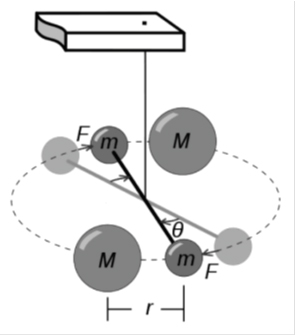Figure 3. Schematic Diagram of the Cavendish Torsion Balance (There are no M Masses): M - Small Masses of The Dumbbell, F - External Forces Acting on M, Ѳ - Angle of Rotation of the Dumbbell under the Action of an External Force

The simplest scheme was used without masses M, and one of the masses was supplemented with a radioactive source (Figure. 3). In principle, for the Pioneers, the generators also form dumbbells, which are acted upon by the flux of solar neutrinos; therefore, the estimates of the forces of influence methodically coincide. The difference is only in the activity of masses and generators, their masses, but this is only for the Earth's zone. As the Pioneers move away from the Earth (Sun), the solar neutrino flux falls in proportion to the square of the distance between the spacecraft and the Sun. Accordingly, the heat flux from the generators and the mechanical pressure (thrust) from the Sun decrease, which, in the absence of understanding the essence of the ANRI effect, is interpreted as a manifestation of the action of an unknown braking force. Therefore, let us consider in detail the interaction of the radioactive mass and the solar neutrino flux. For the first time, the experiment on the influence of the solar neutrino flux on the CTB according to this scheme was carried out from September 7 to October 7, 2015. The number of neutrinos Nv emitted during T = 1 s is completely determined by the solar luminosity erg / s: neutrino / s. Calculations of the neutrino flux for the standard model of the Sun, performed by J. Bakal (USA), give a value of 7.6 ... 3.3 SNU, while the neutrino flux measured at the Davis installation (USA, 1981) with energies above 0.814 MeV is 1, 8 ... 0.3 SNU (see Neutrino Astronomy). The discrepancy between the predicted and measured values ​​can be explained by two general reasons: A) more complex internal processes on the Sun not reflected in the accepted models. These processes can reduce the neutrino flux in the high-energy part of the spectrum. An extreme possibility of this kind is the presence of another source of energy in the sun is not currently recognized. B) the properties of neutrinos. For example, neutrino oscillations, the concept of which was first introduced by B.M. Pontecorvo in 1957, or the decay of ve on the way from the Sun to the Earth. The total number of emitted neutrinos Фγ can be estimated based on the luminosity of the Sun. The number of neutrinos emitted by the Sun is obtained by multiplying the total number of reactions N≈1038 s-1 by two, and their flux to the Earth by dividing it by 4πR2 (where R is the distance of the Earth from the Sun): 6 × 1010 neutrinos / (cm2 × s).

Measurements at CTB. Data on the angle of rotation Ѳ of the beam of the torsion balance were recorded from the device every 30 minutes and entered into the computer. The CTB parameters are as follows (Fig. 3). The oscillation period of the balance is 539 s, the quality factor is 18, the mass of the cargo is 9.62 g, the mass of the radioactive powder is 300 mg. The center of mass of the weights is shifted inward by 0.73 mm. The diameter of the main thread is 100 mm long, 12 microns. The diameter of the auxiliary thread is 50 microns. Its length is 103 mm. Length of cylindrical weights 12 mm, diameter 11.3 mm. The distance between the ends of the cylindrical weights is 82.8 mm. The distance to the centers of the cylinders is 35.4 mm. The copper rocker (dumbbell) has a diameter of 0.8 mm. and a mass of 0.261 g. The distance from the lower point of attachment of the thread to the rocker arm is 74.9 mm. The weight of the suspension is 0.354 g. The moment of inertia of the working medium is 2.3270 × 10–5 kg × m2. Stiffness of the suspension thread κ = 3.1622 × 10-9 J. Modulus of shear of the thread material 155.3 GPa. Distance from mirror to scale 1250 mm. The amplitude of the dumbbell's daily periodicity is 1 mm, that is, ϕ = 1/2500 = 0.0004 rad., and the moment of forces acting on the dumbbell is N = 3.1622 × 10–9 × 4 × 10-4, whence Eabsop. = 1.2649 × 10–12 J. The external force acting on the dumbbell is F, and this force is F = N / 35.4 × 10–3 N = 3.573 × 10–11 N. Since the force acting on the CTB dumbbell arises from the interaction of the neutrino flux only with radioactive ore amounting to 300 mg of 9.62 g or 3.12% of the total mass, we obtain the total force acting on a cylinder with ore weighing 9.62 g, equal to FR = 1.238 × 10-9 N (Newton). According to research, solar neutrinos have a spectrum (Figure. 4).Figure 4. Fluxes of Various Types of Solar Neutrinos Measured On Earth

The average energy of solar neutrinos is ~ 1 MeV, Ес = 6 × 1010 × 1 MeV = 6 * 1010 × 1.6 × 10–13 ~ 10–2 J. However, the neutrino interaction cross section can be determined as the ratio of the energy of the absorbed flux Eabsop. to the solar incident neutrino flux Esol .The incident neutrino flux from the Sun is Еsol ~ 10–2 J / s cm2, Eabsop = 1.2649 × 10–12 J / s cm2, therefore Σore = Eabsop / Esol ~ 1.26 × 10–10, which is significantly higher than the generally accepted conventional estimates (σ = 10–38 - 10–44). These measurements correspond only to the Baikal ore, can be considered as a broadband receiver, probably, the result may differ on other radioactive isotopes. Further, the variations of the dumbbell CTB and Kp indices were analyzed jointly.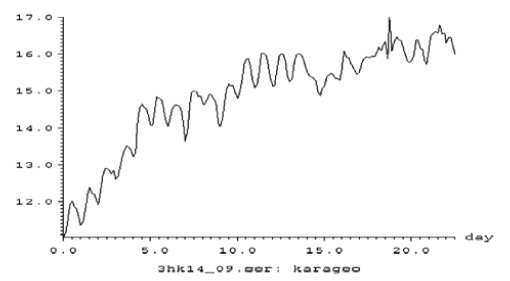Figure 5. Variations in the Rotation of the CTB Dumbbell in Relative Units When Averaged Over 3 Hours Synchronously with Variations in the Kp Indices

The data obtained on the variations in Kp indices and dumbbells were analyzed (Figure. 6). As follows from Figure. 6, in full agreement with the data of R. Davis (Figure. 4), there is a significant anti - correlation (P> 0.999), which is also confirmed by spectral analysis (Figure. 7).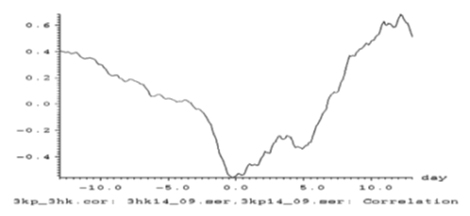Figure 6. Cross-Correlation Function between Variations of the Kp Indices and Perturbations of the Dumbbell Torsion Balance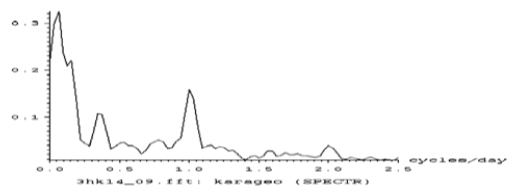Figure 7. The Spectrum of Variations of the Torsion Pendulum (Periods Are Observed: 16, 2.9, 1.0, 0.5 Days)

To compare the digital thermal thermometers, we used the temperature records of the Baikal uranium ore irradiated it with the Cs137 neutrino flux (Figure. 8).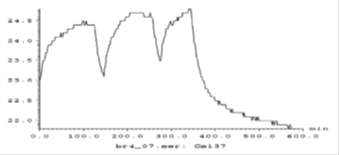Figure 8. Fragment of the Record of Temperature Variations of the Baikal Uranium Ore (Fig. 5) At Irradiation of 20,000 Bq. At A Distance of 2 Cm = 0.02 M

Designations: Bq- Becquerel is the decay unit of a radioactive substance (s); I = irradiation intensity 20,000 Bq. Cs137 with 1 cm = 0.01m thick lead capsule embedded inside. Irradiation time Δt = 3,600 sec = 1 hour, mass of irradiated ore 1 g = 10–3 kg, specific heat capacity of ore Csh (–U235)) = 0.01421 J / 0K, irradiation area S = 1 cm2 = 0.0001 m2, weight = 1 g = 10–3 kg. Eobl = I × CpU × m × Δt 0 × S = {0.01421 J / g0K × 2 × 104 × 4.0 × 103 × 1000 × 1} ~ 4 × 10–2 J. For 3,600 s. the neutrino energy per 1 cm2 will be Eneu = 3 × 10 J, and the ratio Eheat / Eneu = 2.42 × 10–10. This ratio is more than 2 times higher than the previous value obtained with the torsional pendulum Epogl / Esoln = 1.26 × 10–10.

Let us calculate the heating of the ore due to the additional irradiation of 20,000 Bq. Assuming: specific heat Csp (U235) = 0.01421 J / K0, exposure time Δt = 3600 s. [at 1Bq = 1 MeV = 1.6 × 10–13 J, r = 2 cm, S = 1 cm] we have Eobl. = СрU × 20,000 Bq / 4πr2 × Δt × S ~ ~ [0.1 421 × 2 × 104 × 4.0 × 103 × 3,600 × 1] ~ [1.6 × 10–13 × × 1.4 × 1012] ~ 2.2 × 10–1 J. Whence CpU × m × ΔT0 = Eobl ~ 2.2 × 10 –1 / 0.1421 ~ 1.5 0C. Thus, when a neutrino is irradiated with 20,000 Bq for an hour, the temperature should increase by 1.5 0C, which is observed in the experiment.

## Conclusions

1. The Cavendish  torsion balance ( CTB) made it possible to experimentally determine the cross section for the capture of radioactive isotopes under the influence of a solar neutrino flux (~ 10–12), that is, the radioisotope elements of the "Pioneers".
2. CTB is a modern effective device for studying the interaction of neutrino fluxes and radioactive substances and other problems of nuclear and fundamental physics and space technology.
3. Comparison of the capabilities, efficiency and cost of CTB and other modern research facilities (hadron collider, tokamak, neutrino detectors or telescopes, gravitational antennas) leads to the conclusion about the introduction of an economic moratorium on the construction of insanely expensive but sterile research instruments and systems.
4.  The temperature neutrino sensor on the Baikal uranium ore showed the adequacy of the estimates obtained on the torsion pendulum, that is, the braking mechanism of the "Pioneers".
5. The ANRI -effect is applicable in all types of research and technologies, design of modern space technology, as well as when using devices containing isotopes in space, it is necessary to take into account the role of ANRI - effect.
6. In accordance with clause 5, at distances of "Pioneer 10" at 82a.e. and "Pioneer 11" at 43a.u. in proportion to these distances, the value of the ANRI effect fell, which was perceived by observers as a manifestation of the inhibitory force.
7. For a more correct assessment of the fall in the value of the ANRI effect, it is necessary to carry out the above experiments with the use of radioisotopes located on the "Pioneers" and new values ​​of the distances of the vehicles.

## References

1. Bakal J (1993) Neutrino Astrophysics. M.: Mir.
2. Khavroshkin OB, Tsyplakov VV (2013) Radioactivity ore sample: monitoring // Engineering Physics. 8: 53 -62.
3. Khavroshkin O, Tsyplakov V (2013) Sun, Earth, radioactive ore: common periodicity. The Natural Science (NS). 5: 1001-1005.
4. Khavroshkin O, Tsyplakov V (2011) Radioactivity of nuclei in a centrifugal force field. The Natural Science (NS) 3: 733-737.
5. Khavroshkin OB, Tsyplakov VV (2013) Natural radioactivity as an open system // Engineering physics. No. 12.
6. Starodubov AV, Khavroshkin OB, Tsyplakov VV (2014) From periodicities of radioactivity to cosmic and metaphysical oscillations // Metaphysics. 1: 137-149.
7. Khavroshkin OB, Tsyplakov VV (2017) Temperature fields of radioactive substances: time variations and applications // Engineering Physics. 7: 15-31.
8. Karagioz OV, Khavroshkin OB, Tsyplakov VV (2016) Torsion balance of Cavendish and the Zern hadron collider: different destinies and results // Engineering Physics. 4: 3-8.
9. Khavroshkin OB, Tsyplakov VV (2017) Cavendish torsion Balance and Hadron Collider at the Cern: Different Fates and Results. SF J Nucl Sci.
10. Khavroshkin OB, Tsyplakov VV (2018) Devices for advanced physics research. IJRTEM 2: 59-63.
11. Khavroshkin OB, Tsyplakov VV (2017) Temperature fields of radioactive substances: time variations and applications // Engineering Physics 7: 17-32.
12. Khavroshkin OB, Tsyplakov VV (2018) Anomalous Neutrino Radio Isotope Effect (ANRI) absorption: 5 years later // Engineering Physics 7: 14-24.
13. Khavroshkin OB, Tsyplakov VV (2017) Neutrinos: experiments, new results. Palmarium Academic Publishing 265.

Research Article

#### Publication history

Received date: September 08, 2020
Accepted date: September 18, 2020
Published date: September 26, 2020

#### Copyright

©2020 Khavroshkin OB. This is an open-access article distributed under the terms of the Creative Commons Attribution License, which permits unrestricted use, distribution, and reproduction in any medium, provided the original author and source are credited.

#### Citation

Khavroshkin OB, Tsyplakov VV, Georgievich ST (2020) Pioneers Anomaly: Deep Space, ANRI-Effect. OSP J Phy Astronomy 1. JPA-1-104

#### Khavroshkin OB

Institute of Earth physics. RAS Moscow, Russia. khavole@ifz.ruFigure 1. Scheme the Exit of "Pioneers" and "Voyagers" Outside the Solar SystemFigure 2.  Diagram of the "Pioneer-10" apparatus / © wikipedia.orgFigure 3. Schematic Diagram of the Cavendish Torsion Balance (There are no M Masses): M - Small Masses of The Dumbbell, F - External Forces Acting on M, Ѳ - Angle of Rotation of the Dumbbell under the Action of an External ForceFigure 4. Fluxes of Various Types of Solar Neutrinos Measured On EarthFigure 5. Variations in the Rotation of the CTB Dumbbell in Relative Units When Averaged Over 3 Hours Synchronously with Variations in the Kp IndicesFigure 6. Cross-Correlation Function between Variations of the Kp Indices and Perturbations of the Dumbbell Torsion BalanceFigure 7. The Spectrum of Variations of the Torsion Pendulum (Periods Are Observed: 16, 2.9, 1.0, 0.5 Days)Figure 8. Fragment of the Record of Temperature Variations of the Baikal Uranium Ore (Fig. 5) At Irradiation of 20,000 Bq. At A Distance of 2 Cm = 0.02 M